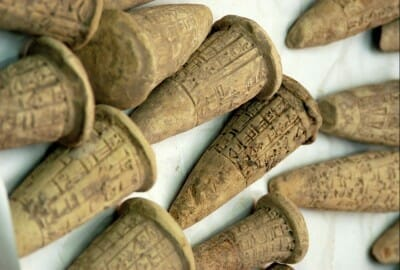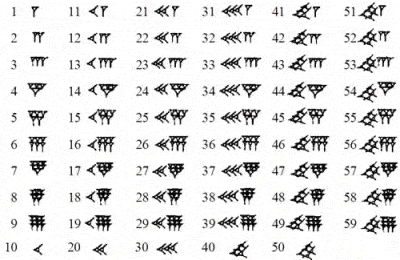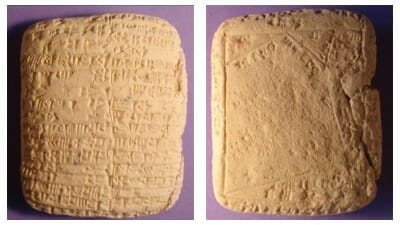# SUMERIAN/BABYLONIAN MATHEMATICSSumerian Clay Cones

Sumer (a region of Mesopotamia, modern-day Iraq) was the birthplace of writing, the wheel, agriculture, the arch, the plow, irrigation and many other innovations, and is often referred to as the Cradle of Civilization. The Sumerians developed the earliest known writing system – a pictographic writing system known as cuneiform script, using wedge-shaped characters inscribed on baked clay tablets – and this has meant that we actually have more knowledge of ancient Sumerian and Babylonian mathematics than of early Egyptian mathematics. Indeed, we even have what appear to school exercises in arithmetic and geometric problems.

As in Egypt, Sumerian mathematics initially developed largely as a response to bureaucratic needs when their civilization settled and developed agriculture (possibly as early as the 6th millennium BCE) for the measurement of plots of land, the taxation of individuals, etc. In addition, the Sumerians and Babylonians needed to describe quite large numbers as they attempted to chart the course of the night sky and develop their sophisticated lunar calendar.

They were perhaps the first people to assign symbols to groups of objects in an attempt to make the description of larger numbers easier. They moved from using separate tokens or symbols to represent sheaves of wheat, jars of oil, etc, to the more abstract use of a symbol for specific numbers of anything.

Starting as early as the 4th millennium BCE, they began using a small clay cone to represent one, a clay ball for ten, and a large cone for sixty. Over the course of the third millennium, these objects were replaced by cuneiform equivalents so that numbers could be written with the same stylus that was being used for the words in the text. A rudimentary model of the abacus was probably in use in Sumeria from as early as 2700 – 2300 BCE.

## Sumerian & Babylonian Number System: Base 60Babylonian Numerals

Sumerian and Babylonian mathematics was based on a sexegesimal, or base 60, numeric system, which could be counted physically using the twelve knuckles on one hand the five fingers on the other hand. Unlike those of the Egyptians, Greeks and Romans, Babylonian numbers used a true place-value system, where digits written in the left column represented larger values, much as in the modern decimal system, although of course using base 60 not base 10. Thus,in the Babylonian system represented 3,600 plus 60 plus 1, or 3,661. Also, to represent the numbers 1 – 59 within each place value, two distinct symbols were used, a unit symbol () and a ten symbol () which were combined in a similar way to the familiar system of Roman numerals (e.g. 23 would be shown as). Thus,represents 60 plus 23, or 83. However, the number 60 was represented by the same symbol as the number 1 and, because they lacked an equivalent of the decimal point, the actual place value of a symbol often had to be inferred from the context.

It has been conjectured that Babylonian advances in mathematics were probably facilitated by the fact that 60 has many divisors (1, 2, 3, 4, 5, 6, 10, 12, 15, 20, 30 and 60 – in fact, 60 is the smallest integer divisible by all integers from 1 to 6), and the continued modern-day usage of of 60 seconds in a minute, 60 minutes in an hour, and 360 (60 x 6) degrees in a circle, are all testaments to the ancient Babylonian system. It is for similar reasons that 12 (which has factors of 1, 2, 3, 4 and 6) has been such a popular multiple historically (e.g. 12 months, 12 inches, 12 pence, 2 x 12 hours, etc).

The Babylonians also developed another revolutionary mathematical concept, something else that the Egyptians, Greeks and Romans did not have, a circle character for zero, although its symbol was really still more of a placeholder than a number in its own right.

## Babylonian Clay tablets

We have evidence of the development of a complex system of metrology in Sumer from about 3000 BCE, and multiplication and reciprocal (division) tables, tables of squares, square roots and cube roots, geometrical exercises and division problems from around 2600 BCE onwards. Later Babylonian tablets dating from about 1800 to 1600 BCE cover topics as varied as fractions, algebra, methods for solving linear, quadratic and even some cubic equations, and the calculation of regular reciprocal pairs (pairs of number which multiply together to give 60). One Babylonian tablet gives an approximation to √2 accurate to an astonishing five decimal places. Others list the squares of numbers up to 59, the cubes of numbers up to 32 as well as tables of compound interest. Yet another gives an estimate for π of 3 18 (3.125, a reasonable approximation of the real value of 3.1416).Babylonian Clay tablets from c. 2100 BCE showing a problem concerning the area of an irregular shape

The idea of square numbers and quadratic equations (where the unknown quantity is multiplied by itself, e.g. x2) naturally arose in the context of the meaurement of land, and Babylonian mathematical tablets give us the first ever evidence of the solution of quadratic equations. The Babylonian approach to solving them usually revolved around a kind of geometric game of slicing up and rearranging shapes, although the use of algebra and quadratic equations also appears. At least some of the examples we have appear to indicate problem-solving for its own sake rather than in order to resolve a concrete practical problem.

The Babylonians used geometric shapes in their buildings and design and in dice for the leisure games which were so popular in their society, such as the ancient game of backgammon. Their geometry extended to the calculation of the areas of rectangles, triangles and trapezoids, as well as the volumes of simple shapes such as bricks and cylinders (although not pyramids).

### Plimpton 322 clay tablet

The famous and controversial Plimpton 322 clay tablet, believed to date from around 1800 BCE, suggests that the Babylonians may well have known the secret of right-angled triangles (that the square of the hypotenuse equals the sum of the square of the other two sides) many centuries before the Greek Pythagoras. The tablet appears to list 15 perfect Pythagorean triangles with whole number sides, although some claim that they were merely academic exercises, and not deliberate manifestations of Pythagorean triples.